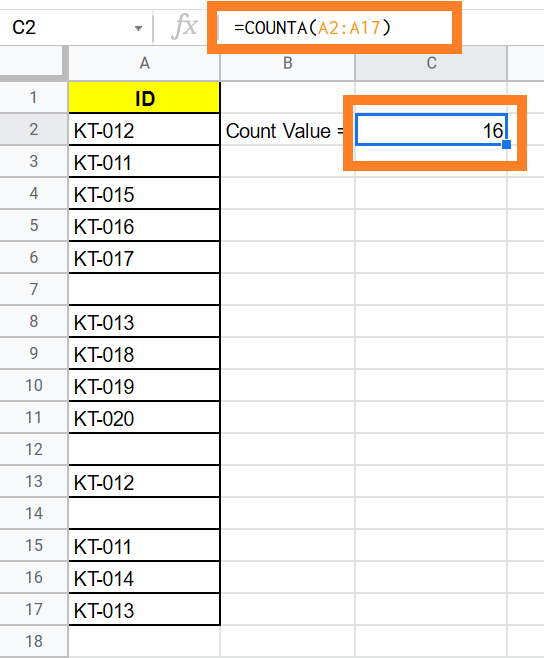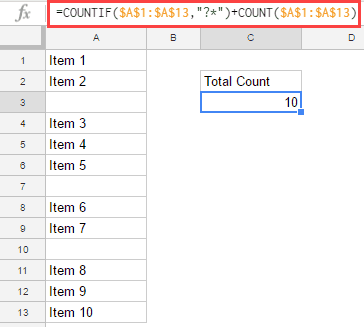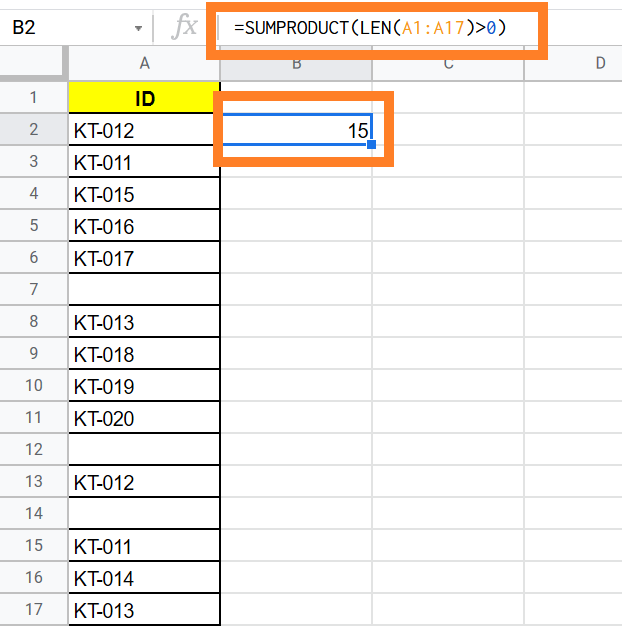# How to Count Cells If Not Blank in Google Sheets

Google Sheets If Not Blank: It is really difficult for one to find the cells which are not blank  in the numerous datasets mannually. To find the non blank cells in the given dataset on Google sheet, one can make use of Google Sheet count functions. The Count functions of the Google sheet will help us count the non-blank cells. In this article let’s discuss everything about Google sheets Count Empty cells function in detail.

Also check our page Google Sheet Tips & Tricks which will help you with various formulas to learn features of Google Sheet like a pro.

## Count Cells If Not Blank Using COUNTA Function

In Google Sheets, COUNTA function counts all cells with a certain value or text string and does not take into account the cells that are blank.

Let’s consider that you have the following dataset. Now you will have to count the cells which are not blank using COUNTA.

Google Sheets IF Not Blank Steps

• Step 1: Select the cell range where you will have to count the non blank cells.
• Step 2: Now type the formula “=COUNTA(Cell Range)
• Step 3: Press “Enter”.

The results will be displayed on the screen as shown below.While COUNTA functions work most of the time, it fails to fetch accurate results if the cell contains the “Empty Strings” or “Apostrophe”.

In the above example, the actual result should 14. But, since the “Apostrophe” is present in cells A7 & A12, the count is showing as 16.Hence to overcome this and to find the accurate non-blank cells, we can use the COUNTIF function which gives accuarte results.

### Count Cells If Not Blank Using COUNTIF Function

COUNTIF function on the Google sheets find the non empty cells when certain conditions are met. One can use the formula “=COUNTIF(\$A\$1:\$A\$13,”?*”)+COUNT(\$A\$1:\$A\$13)” to find the cells that are blank.

Let’s understand how the COUNTIF function works with an example now.

• Step 1: Select the cell range to count the non-empty cells.
• Step 2: Now enter the formula “=COUNTIF(\$A\$1:\$A\$13,”?*”)+COUNT(\$A\$1:\$A\$13)
• Step 3: Click on “Enter” button.
• Step 4: You will find the accurate count.If you see the results here, the COUNTIF function has ignored the “Empty Strings” or “Apostrophe” and resulted the correct answer.

### Count Cells IF Not Blank Using SUMPRODUCT Formula

Other easy method to check if the cell is not empty is to apply SUMPRODUCT formula. Follow the steps listed below to apply the SUMPRODUCT Formula for Google sheets count if cell contains text:

• Step 1: Select the cell range.
• Step 2: Enter the formula =SUMPRODUCT(LEN(First Cell Value:Last Cell Value)>0)), i.e., =SUMPRODUCT(LEN(A1:A17)>0)).
• Step 3: Press “Enter“.The Google sheets gives you the count of the cells which consists the text.

However this method is not reliable since it counts the value of “Empty Strings” or “Apostrophe” as well.